Courses

# Circuit Theorems - 3

## 20 Questions MCQ Test Topicwise Question Bank for Electrical Engineering | Circuit Theorems - 3

Description
This mock test of Circuit Theorems - 3 for Electrical Engineering (EE) helps you for every Electrical Engineering (EE) entrance exam. This contains 20 Multiple Choice Questions for Electrical Engineering (EE) Circuit Theorems - 3 (mcq) to study with solutions a complete question bank. The solved questions answers in this Circuit Theorems - 3 quiz give you a good mix of easy questions and tough questions. Electrical Engineering (EE) students definitely take this Circuit Theorems - 3 exercise for a better result in the exam. You can find other Circuit Theorems - 3 extra questions, long questions & short questions for Electrical Engineering (EE) on EduRev as well by searching above.
QUESTION: 1

### The current flowing through the resistance of 10 Ω for the circuit shown below is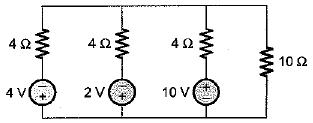Solution:

The given circuit can be replaced by an equivalent circuit as shown below: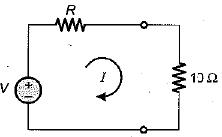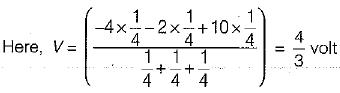and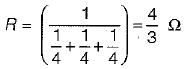Hence,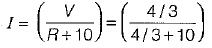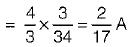QUESTION: 2

### The Thevenin’s equivalent voltage source across the terminals X-Y shown below is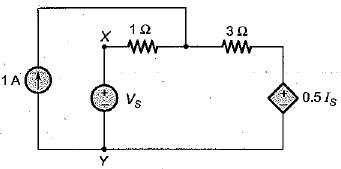Solution:

For finding Voc' the terminal X-Y is open circuited. Thus, current through voltage source (\/s) becomes zero. i.e. Is = 0 due to which 0.5Is voltage source gets short circuited.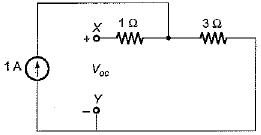Thus, Voc = 3 x 1 = 3 volt

QUESTION: 3

### The Norton’s equivalent resistance across the diode in the given circuit is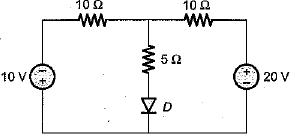Solution:

For finding RN across diode, the independent voltage sources will be short circuited.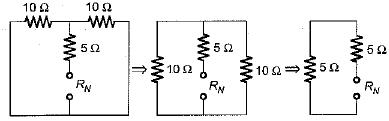Hence, RN = 5 + 5 = 10 Ω

QUESTION: 4

Assuming the op-amp to be ideal, the input admittance of the op-amp shown below is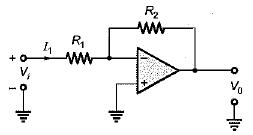Solution:

Since the op-amp is ideal, the inverting terminal will be virtually grounded.
Hence, Vi = I1 R1
or,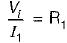or,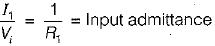QUESTION: 5

In the π-circuit shown below, the current through the 2 Ω, resistor is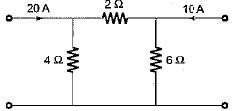Solution:

Here, we apply superposition theorem.
Taking the 20 A current source only: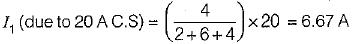Taking the 10 A current source only: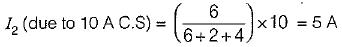Hence, the net current through the 2 Ω resistor
= I- I2 = (6.67 - 5) A = 1.67A

QUESTION: 6

Match List-I (Elements) with List-ll (Type) and select the correct answer using the codes given below the lists: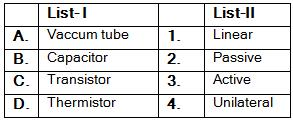Codes: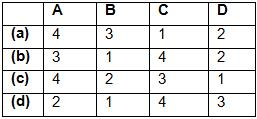Solution:
QUESTION: 7

The equivalent resistance of the given circuit is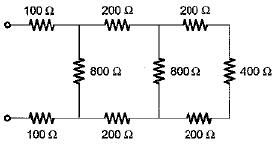Solution:

Given circuit can be reduced as shown below.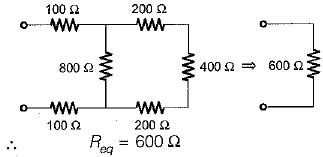QUESTION: 8

The maximum power that a 12 V d.c. source with an interna! resistance of 2 Ω can supply toa resistive load is

Solution: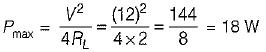QUESTION: 9

The Norton’s equivalent circuit for the circuit shown below across the terminals X-Y can be represented as a current source (IN) connected across a resistance (RN). The value of IN and RN are respectively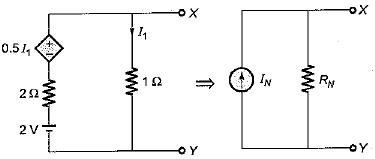Solution:

Here, VX-Y = VOC = 1 x I1 = I1
Applying KVL in the loop, we have
I1 x 1 - 2 + 2 I- 0.5 I1 = 0
or, I1 - 0.8 A
Hence, VOC = 0.8 V
When the termical X-Y is short circuited then,
I1 = 0
∴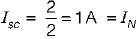So,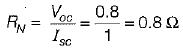QUESTION: 10

To effect maximum power transfer to the load, ZL (W) for the circuit shown below should be​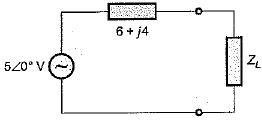Solution:

For maximum power transfer,
ZL = ZS*
or, ZL = 6 - j4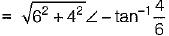or, ZL = 7.211 ∠-33°.69Ω

QUESTION: 11

In order to apply superposition theorem, it is necessary that the network be only

Solution:
QUESTION: 12

A network N is to be connected to a load of 500 ohms. If the Thevenin’s equivalent voltage and Norton’s equivalent current of N are 5 volts and 10 mA respectively, the current through the load will be

Solution:

Given,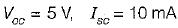∴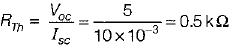= 500 Ω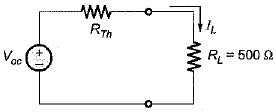Hence, the current through the load is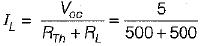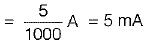QUESTION: 13

If all the elements in a particular network are linear, then the superposition theorem would hold, when the excitation is

Solution:
QUESTION: 14

The maximum value of power transferred to the load ZL shown in figure below is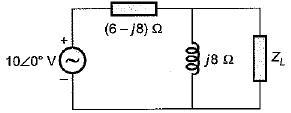Solution: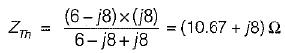Also, VOC across ZL is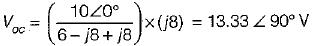According to maximum power transfer theorem,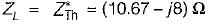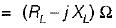Hence,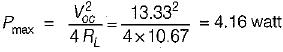QUESTION: 15

In the circuit shown below, the current through the inductive reactance is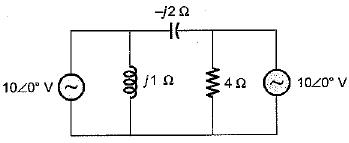Solution:

Let us apply superposition theorem.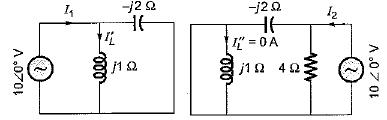Here,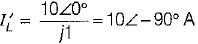and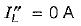∴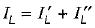= 10 ∠- 90 ° + 0 = -j10 A

QUESTION: 16

Which of the following theorems is applicable for both linear and non-linear circuits?

Solution:
QUESTION: 17

While determining RTh of a circuit
1. voltage and current sources should be left as they are.
2. all independent current and voltage sources are short-circuited.
3. all sources should be replaced by their source resistances.
4. all dependent sources remain as they are.
Q. Which of the statements is/are true in relation of RTh?

Solution:
QUESTION: 18

For the circuit given in figure below, the Thevenin’s voltage and resistance as seen at “AB" are respectively​

Solution: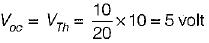(Using voltage divider rule)
RTh across,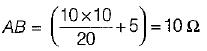QUESTION: 19

Consider the following statements about Norton’s equivalent of a circuit across a given two load terminals:
1. Norton’s equivalent resistance is the same as Thevenin’s equivalent resistance RTh.
2. Norton’s equivalent is the voltage equivalent of the network.
3. The load is connected in parallel to the Norton’s equivalent resistance.
Out of the above statements, which statements hold true?

Solution:
QUESTION: 20

When the power transferred to the load is maximum, the efficiency is

Solution: